medfilt1

1-D median filtering

Description

y = medfilt1(x) applies a third-order one-dimensional median filter to the input vector, x. The function considers the signal to be 0 beyond the endpoints. The output, y, has the same length as x.

example

y = medfilt1(x,n) applies an nth-order one-dimensional median filter to x.

y = medfilt1(x,n,blksz,dim) or y = medfilt1(x,n,[],dim) specifies the dimension, dim, along which the filter operates. blksz is required for backward compatibility and is ignored.

example

y = medfilt1(___,nanflag,padding) specifies how NaN values are treated over each segment, using any input arguments from previous syntaxes. This syntax also specifies padding, the type of filtering performed at the signal edges.

nanflag and padding can appear anywhere after x in the function call.

Examples

collapse all

Generate a sinusoidal signal sampled for 1 second at 100 Hz. Add a higher-frequency sinusoid to simulate noise.

fs = 100;
t = 0:1/fs:1;
x = sin(2*pi*t*3)+0.25*sin(2*pi*t*40);

Use a 10th-order median filter to smooth the signal. Plot the result.

y = medfilt1(x,10);

plot(t,x,t,y)
legend('Original','Filtered')
legend('boxoff')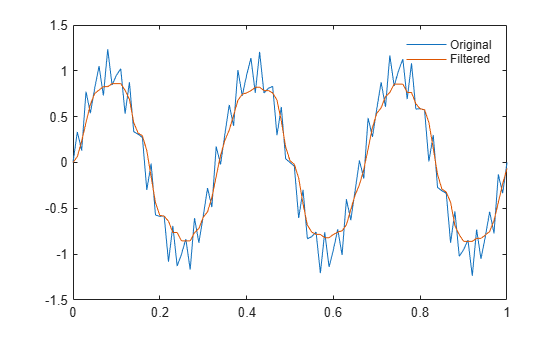Generate a two-channel signal consisting of sinusoids of different frequencies. Place spikes in random places. Use NaNs to add missing samples at random. Reset the random number generator for reproducible results. Plot the signal.

rng('default')

n = 59;
x = sin(pi./[15 10]'*(1:n)+pi/3)';

spk = randi(2*n,9,1);
x(spk) = x(spk)*2;
x(randi(2*n,6,1)) = NaN;

plot(x)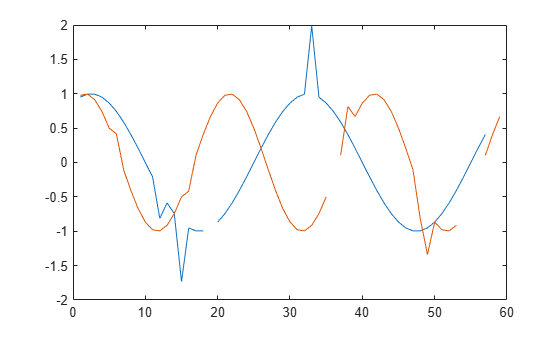Filter the signal using medfilt1 with the default settings. Plot the filtered signal. By default, the filter assigns NaN to the median of any segment with missing samples.

y = medfilt1(x);
plot(y)Transpose the original signal. Filter it again, specifying that the function work along the rows. Exclude the missing samples when computing the medians. If you leave the second argument empty, then medfilt1 uses the default filter order of 3.

y = medfilt1(x',[],[],2,'omitnan');
plot(y')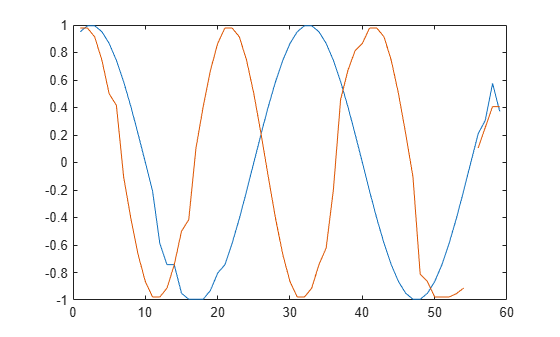The function cannot assign a value to the segment that contains only NaNs. Increase the segment length to fix this issue. The change also removes the outlier more thoroughly.

y = medfilt1(x,4,'omitnan');
plot(y)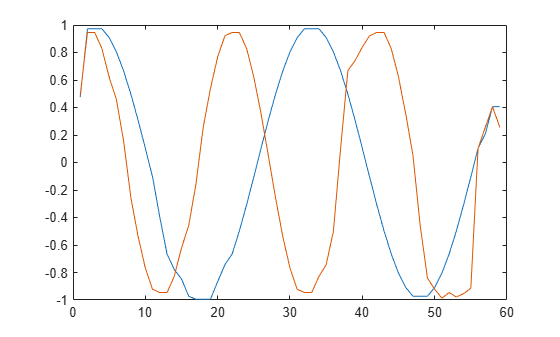The default zero padding results in the function's underestimating the signal values at the edges. Lessen this effect by using decreasing windows to compute the medians at the ends.

y = medfilt1(x,4,'omitnan','truncate');
plot(y)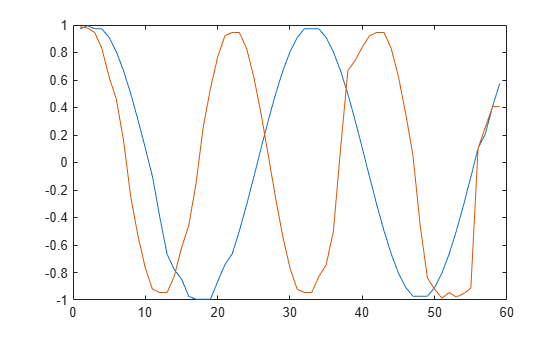Input Arguments

collapse all

Input signal, specified as a real-valued vector, matrix, or N-D array.

Data Types: single | double

Order of the one-dimensional median filter, specified as a positive integer scalar.

• When n is odd, y(k) is the median of x(k-(n-1)/2:k+(n-1)/2).

• When n is even, y(k) is the median of x(k-n/2:k+(n/2)-1). In this case, medfilt1 sorts the numbers and takes the average of the two middle elements of the sorted list.

Example: If n = 11, then y(k) is the median of x(k-5:k+5).

Example: If n = 12, then y(k) is the median of x(k-6:k+5).

Data Types: double

Dimension to filter along, specified as a positive integer scalar. By default, medfilt1 operates along the first nonsingleton dimension of x. In particular, if x is a matrix, the function filters its columns so that y(:,i) = medfilt1(x(:,i),n).

Data Types: double

NaN condition, specified as 'includenan' or 'omitnan'.

• 'includenan' — Returns the filtered signal so that the median of any segment containing NaNs is also NaN.

• 'omitnan' — Returns the filtered signal so that the median of any segment containing NaNs is the median of the non-NaN values. If all elements of a segment are NaNs, the result is NaN.

Endpoint filtering, specified as 'zeropad' or 'truncate'.

• 'zeropad' — Considers the signal to be zero beyond the endpoints.

• 'truncate' — Computes medians of smaller segments as it reaches the signal edges.

Output Arguments

collapse all

Filtered signal, returned as a real-valued vector, matrix, or N-D array. y is the same size as x

Data Types: double

Tips

If you have a license for Image Processing Toolbox™ software, you can use the medfilt2 (Image Processing Toolbox) function to perform two-dimensional median filtering.

 Pratt, William K. Digital Image Processing. 4th Ed. Hoboken, NJ: John Wiley & Sons, 2007.